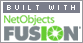Coming to Statistica
in 2022/2023

Zoom tutorials
using Explaineverything app
We a record sessions for you to listen to later

CALL STATISTICA NOW

.

E-mail     anne@statistica.com.au

# Place         Willetton, Western Australia# Mathematics & Statistic Tutor Perth - SPSS Help# Exponential Growth and Decay

N(t) =  N e kt

General form though we could have a number where we have ‘e’.
Here the growth or decay is not linear
ie it does not increase or decrease by the same amount each time.
The growth or decay increases or decreases by multiples of an amount
ie if it doubles each second and you start with 3 units then after 4 seconds, it would be 48 units
The equation for this situation would be

N(t) = 3 (2) t

These questions are not too difficult to solve we just need to be careful.  There are several possible questions that can be asked.

## K is the Growth or Decay Factor

Finding k or t uses the same method,
so once you know how to complete one
the other is just as easy

# Exponential Growth Example

If a colony of ants starts with a population of 25 000 then after 10 hours there are 40 000 ants.
If the population follows an exponential growth of
Q(t) =  Qokt
Find the value of k
and
when the population exceeds 60 000 ants#[Home] [Parents] [Contact us] [Services] [Mathematics] [Statistics] [School]